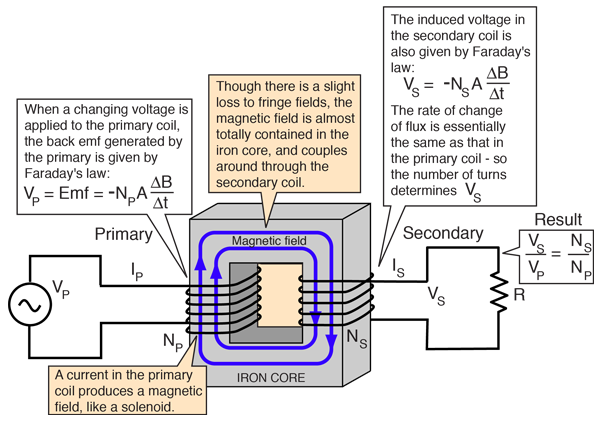# Transformer

A transformer makes use of Faraday's law and the ferromagnetic properties of an iron core to efficiently raise or lower AC voltages. It of course cannot increase power so that if the voltage is raised, the current is proportionally lowered and vice versa.ShowCalculation
Index

Transformer concepts

Magnetic field concepts

 HyperPhysics*****Electricity and magnetism R Nave
Go BackFaraday's law Solenoid magnetic field Calculation
Index

Magnetic force

Transformer concepts

 HyperPhysics*****Electricity and magnetism R Nave
Go Back

# Ideal Transformer Calculation

Primary
 Primary voltage = VP = volts.
 Primary current = IP = amps
 Primary turns =NP =turns
Secondary
 Secondary voltage = VS = volts
 Secondary current = IS = amps
 Secondary turns = NS = turns
 Load resistor = R = ohms
For an ideal transformer:Power
 Power used = PP = PS = watts

The ideal transformer neglects losses to resistive heating in the primary coil and assumes ideal coupling to the secondary (i.e., no magnetic losses).

 More realistic treatment: Numerical example Calculation with primary losses

Notes: For this exploratory calculation, you may enter data for any parameter except the power. Then click on the active text for the parameter you wish to calculate; values will not be forced to be consistent until you do. For primary calculations, the voltage and number of turns in the secondary will be considered to be established and vice versa. Default values will be entered for unspecified parameters, but all values except the power may be changed.

Index

Transformer concepts